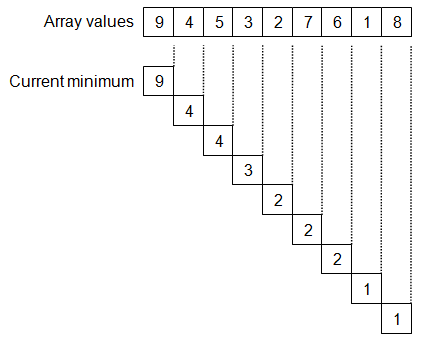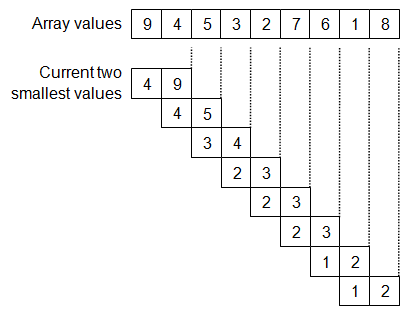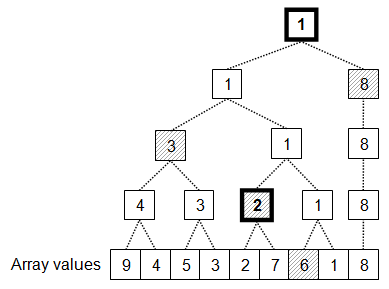# Finding Two Smallest Numbers in an Array

by Zoran Horvat

## Problem Statement

Given the array of N integers (N > 1), write the function that finds the two smallest numbers.

Example: Suppose that array is: 9, 4, 5, 3, 2, 7, 6, 1, 8. Two smallest values in the array are 1 and 2.

Keywords: Array, two smallest, minimum.

## Problem Analysis

Finding smallest element in an array is fairly straight-forward: just traverse the array, keeping the current minimum value; every time when an element is encountered that has value smaller than current minimum, simply update the current minimum and continue further. Once the end of the array is reached, current minimum will be the overall minimum of the array. Initially, the first element of the array is taken as the minimum. Should the array have only one element, that element would naturally be the overall minimum. The following picture shows the process of selecting minimum value from an example array. This solution requires N-1 comparisons for an array of N elements.Looking for the second smallest value means just to track two minimum values: the overall minimum and the runner-up. Off course, we need to take first two values in the array as initial values for the current minimums pair, but everything else remains almost the same. This process is demonstrated on the following picture.The process of selecting two values rather than one goes by iterating through the rest of the array (skipping the leading two values) and trying to insert each value into the two entries that are kept aside. In the example above, initial pair was (4, 9). Once value 5 has been encountered, value 9 drops out. Same thing happens to value 5 as soon as value 3 has been reached. And so the process goes on, until all values in the array have been exhausted leaving (1, 2) as the final pair of smallest values from the array.

We can now estimate number of comparisons required to solve the problem using this algorithm. Initializing the pair requires one comparison just to find whether the first element is smaller than the second or vice versa. Further on, for the remaining N-2 elements, we have one or two comparisons (with two comparisons being the worst case) to find out whether the current element should stick into the current smallest pair and, if yes, on which position. This makes total of 2N-1 comparisons in the worst case and constant space to solve the problem.

Out of 2N-1 comparisons, N-1 was spent to find the smallest value and additional N comparisons to find the second smallest. This raises the question whether there is a better way to find the runner-up? And there is one: it is solution to the problem known for ages, and it has to do with tennis tournaments. The question was, knowing the outcome of the tennis tournament, how can we tell which player was the second best? The defeated finalist is a good candidate, but there are other players that were defeated directly by the tournament winner and any of them could also be a good candidate for the second best. So the solution to the problem is quite simple: Once the tournament finishes, pick up the logN competitors that were beaten by the tournament winner and hold a mini-tournament to find which one is the best among them. If we imagine that better players correspond with smaller numbers, the algorithm now goes like this. Hold the tournament to find the smallest number (requires N-1 comparisons). During this step, for each number construct the list of numbers it was smaller than. Finally, pick the list of numbers associated with the smallest number and find their minimum in logN-1 steps. This algorithm requires N+logN-2 comparisons to complete, but unfortunately it requires additional space proportional to N (each element except the winner will ultimately be added to someone’s list); it also requires more time per step because of the relatively complex enlisting logic involved in each comparison. When this optimized algorithm is applied to example array, we get the following figure.Tournament held among numbers promotes value 1 as the smallest number. That operation, performed on an array with nine numbers, requires exactly eight comparisons. While promoting the smallest number, this operation has also flagged four numbers that were removed from competition by direct comparison with the future winner: 6, 2, 3 and 8 in that order. Another sequence of three comparisons is required to promote number 2 as the second-smallest number in the array. This totals 11 comparisons, while naive algorithm requires 17 comparisons to come up with the same result.

All in all, this algorithm that minimizes number of comparisons looks to be good only for real tournaments, while number cracking algorithms should keep with the simple logic explained above. Implementation of simple algorithm may look like this:

``````a – array containing n elements
min1 = a – candidate for the smallest value
min2 = a – candidate for the second smallest value

if min2 < min1
min1 = a
min2 = a

for i = 2 to n – 1
if a[i] < min1
min2 = min1
min1 = a[i]
else if a[i] < min2
min2 = a[i]
``````

## Implementation

Here is the of the TwoSmallestValues function, with surrounding testing code.

``````using System;

namespace TwoSmallestNumbers
{

public class Program
{

static Random _rnd = new Random();

static int[] Generate(int n)
{

int[] a = new int[n];

for (int i = 0; i < n; i++)
a[i] = _rnd.Next(100);

return a;

}

static void TwoSmallestValues(int[] a, out int min1, out int min2)
{

min1 = a;
min2 = a;
if (min2 < min1)
{
min1 = a;
min2 = a;
}

for (int i = 2; i < a.Length; i++)
if (a[i] < min1)
{
min2 = min1;
min1 = a[i];
}
else if (a[i] < min2)
{
min2 = a[i];
}

}

static void Main(string[] args)
{

while (true)
{

Console.Write("n=");
int n = int.Parse(Console.ReadLine());

if (n < 2)
break;

int[] a = Generate(n);

int min1, min2;

TwoSmallestValues(a, out min1, out min2);

Console.Write("{0,4} and {1,4} are smallest in:", min1, min2);
for (int i = 0; i < a.Length; i++)
Console.Write("{0,4}", a[i]);
Console.WriteLine();
Console.WriteLine();

}

Console.Write("Press ENTER to continue... ");

}
}
}
``````

## Demonstration

Here is the output produced by the test application:

```            ```
n=10
16 and   41 are smallest in:  44  85  41  95  70  81  77  70  16  52

n=10
0 and   16 are smallest in:  87  27  89  16  55  62  34  18  63   0

n=10
9 and   17 are smallest in:  68  29  89  49  89  17  19  57  33   9

n=17
6 and    6 are smallest in:  38  94  58  65   9  15  95  91   6  53  62  19  37   6  28  77  79

n=5
5 and   10 are smallest in:  10  28   5  64  13

n=3
28 and   35 are smallest in:  35  58  28

n=2
51 and   59 are smallest in:  51  59

n=2
70 and   77 are smallest in:  77  70

n=0
Press ENTER to continue...
```
```

## Follow-Up Exercises

Readers are suggested to build upon this solution and work up solutions to extended tasks:

• Implement solution with reduced number of comparisons.
• Compare time and space requirements for the two solutions.